# First Year Wilkinson Microwave Anisotropy Probe (WMAP) Observations: Parameter Estimation Methodology

## L. Verde, et al., 2003, ApJS, 148, 195

### reprint (423 Kb) / preprint (402 Kb) / individual figures / ADS / astro-ph

 WMAP First-year Paper Figures, Verde, et al. Individual figures are provided for use in talks. Proper display of PNG transparency in PowerPoint requires saving files to your computer before Inserting them. Please acknowledge the WMAP Science Team when using these images. Image Credit: NASA / WMAP Science Team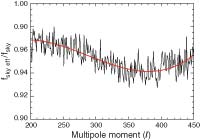" Fig.1Ratio of the effective sky coverage to the actual sky coverage. This correction factor calibrates the expression for the Fisher matrix to the value obtained from the Monte Carlo approach. Here we show the ratio obtained from 100,000 simulations ( jagged line); the smooth curve shows the fit we use, eq. (16). Note that, since we are switching between weighting schemes, the correction factors are not expected to smoothly interpolate between regimes. PNG (33 kb - 1433x2048 pixels) PNG (79 kb - 1433x2048 pixels) EPS (13 kb)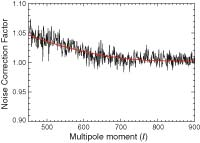" Fig.2Correction factor for the noise. The lines are as in Fig. 1. Note that, since we are switching between weighting schemes, the correction factors are not expected to smoothly interpolate between regimes. PNG (24 kb - 1464x2048 pixels) PNG (61 kb - 2928x4096 pixels) EPS (16 kb)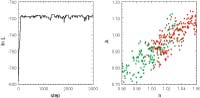" Fig.3Unconverged Markov chains. The left-hand panel shows a trace plot of the likelihood values vs. iteration number for one MCMC (these are the first 3000 steps from one of our ΛCDM model runs). Note the burn-in for the first ~100 steps. In the right-hand panel, red dots are points of the chain in the (n, A)-plane after discarding the burn-in. Green dots are from another MCMC for the same data set and the same model. It is clear that, although the trace plot may appear to indicate that the chain has converged, it has not fully explored the likelihood surface. Using either of these two chains at this stage will give incorrect results for the best-fit cosmological parameters and their errors. PNG (22 kb - 1002x2048 pixels) PNG (52 kb - 2005x4096 pixels) EPS (70 kb)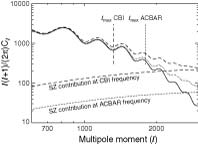" Fig.4CMB angular power spectrum (in µK2) for our best-fit ΛCDM model for l > 800 and the Sunyaev-Zeldovich contribution for σ8 = 0.98 for CBI wavelengths (dotted line) and for ACBAR (dashed line). The vertical line shows the adopted cutoff for CBI and ACBAR. PNG (29 kb - 2091x4096 pixels) PNG (69 kb - 2091x4096 pixels) EPS (22 kb)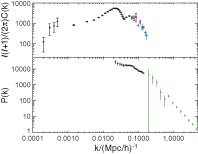" Fig.5Combined CMB and LSS data set. Top: CMB angular power spectrum in µK2 as a function of k, where k is related to l by l = η0k (where η0 ~ 14,400 Mpc is the distance to the last scattering surface). Black points are the WMAP data, red points CBI, blue points ACBAR. Bottom: LSS data. Black points are the 2dFGRS measurements, and green points are the Lyα measurements. Both LSS power spectra are in units of (Mpc h-1)3 and have been rescaled to z = 0. This plot only illustrates the scale coverage of all the data sets we consider. The various LSS power spectra as plotted here cannot be directly compared with the theory because of the effects outlined in § 5 (e.g., redshift-space distortions, nonlinearities, bias and window function effect). PNG (21 kb - 1594x2049 pixels) PNG (51 kb - 3187x4096 pixels) EPS (32 kb)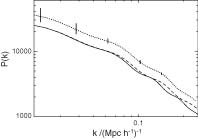" Fig.6Matter power spectrum in (Mpc h-1)3, linear in real space (solid line), nonlinear in real space (dashed line), and nonlinear in redshift space (dotted line). The error bars on the dotted line show the size of the statistical error bars per k bin of the 2dFGRS galaxy power spectrum. PNG (16 kb - 1438x2048 pixels) PNG (41 kb - 2877x4096 pixels) EPS (8 kb)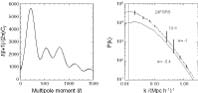" Fig.7Two cosmological models: ωb = 0.0235, ωm = 0.143, ns = 0.978, τ = 0.11, w = -0.426, h = 0.53 (solid line) and ωb = 0.0254, ωm = 0.137, ns = 1.024, τ = 0.08, w = -1, h = 0.77 (dotted line). The two models are indistinguishable within current error bars from the CMB angular power spectrum (left-hand panel; units for the power spectrum are µK2). However, they can easily be distinguished if we can relate the observed power spectrum to the underlying matter power spectrum [right-hand panel; units for the power spectrum are (Mpc h-1)3]. The error bars on the solid line are examples of the size of the 2dFGRS and Lyα power spectra statistical error bars for one data point at different scales. There are four error bars for 2dFGRS and four for Lyα. PNG (21 kb - 957x2048 pixels) PNG (51 kb - 1914x4096 pixels) EPS (22 kb)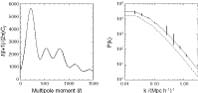" Fig.8Two cosmological models: Ωm = 0.26, ωb = 0.02319, τ = 0.12, ns = 0.953, ωv = 0, h = 0.714 (solid line) and Ωm = 0.26, ωb = 0.02319, τ = 0.12, ns = 0.953, ωv = 0.02, h = 0.6 (dashed line). As before the two models are virtually indistinguishable from the CMB angular power spectrum (left-hand panel; units for the power spectrum are µK2), but they can easily be distinguished if the matter power spectrum amplitude is known [right-hand panel; units for the power spectrum are (Mpc h-1)3]. The error bars on the solid line are examples of the size of the 2dFGRS and Lyα power spectra statistical error bars for one data point. There are four error bars for 2dFGRS and four for Lyα . PNG (20 kb - 957x2048 pixels) PNG (47 kb - 1914x 4096 pixels) EPS (21 kb)
A service of the HEASARC and of the Astrophysics Science Division at NASA/GSFCHEASARC Director: Dr. Alan P. Smale
LAMBDA Director: Dr. Eric R. Switzer
NASA Official: Dr. Eric R. Switzer
Web Curator: Mr. Michael R. Greason